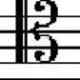#### [SOLVED] Do variations of the Earth's magnetic field affect gravitational acceleration at Earth's surface, and if yes, by how much?

By DeltaIV

The Earth's magnetic field varies with time over geological time scales (we have phenomena such as pole inversion). Can this affect the acceleration of gravity at Earth's surface? I would think that since the magnetic field has an energy density, such energy density affect the gravitational effects of the magnet which generates it (the liquid metal currents inside the Earth). However, even if this is correct, I would expect the effect to be extremely small. So, is there such an effect, and if yes, what's the order of magnitude? 1/10^6 $$g$$? 1/10^9 $$g$$? Etc.#### @G. Smith 2019-02-11 06:46:18

The effect of variations of Earth's magnetic field on gravitational acceleration is completely negligible, as the following rough estimate shows.

The first question is what to take as the magnetic field inside the Earth. Outside, a dipole field is a good approximation, but if we continue to use that field inside, the field strength gets stronger and stronger as we go toward the center. The field strength goes as $$1/r^3$$ and the energy density as this square of this, so $$1/r^6$$. Integrating this over the interior of the Earth gives a divergent integral for the energy in the field.

But the Earth's magnetic field is not really the field of a point dipole at the center of the Earth. Instead it's the field of a messy, rotating, convecting, liquid dynamo the size of the Earth's core. Pictures of it usually make the field lines look like spaghetti. So let's not try to actually model the field inside the Earth. Let's take a very crude estimate.

This article says that the field strength averaged over the outer core is 25 Gauss (0.0025 Teslas), or about 50 times stronger than the field at the surface. Let's just assume that this is the average throughout the interior of the Earth. Maybe the field is stronger in the inner core, but it's definitely weaker outside the outer core, so we're probably doing an overestimate of the effect. But it's going to turn out tiny anyway.

Using this average field strength, the magnetic energy density $$B^2/2\mu_0$$ avereages 2.5 Joules per cubic meter.

The radius of the Earth is $$6.4\times10^6$$ meters, so the volume is $$1.1\times10^{21}$$ cubic meters.

Multiplying the energy density by the volume gives a magnetic energy inside the Earth of $$2.7\times10^{21}$$ Joules.

Dividing by the speed of light squared gives an equivalent mass of 30,000 kilograms.

The mass of the Earth is $$6.0\times10^{24}$$ kilograms, so the mass-equivalent of the magnetic energy comprises only a fraction $$5.0\times10^{-21}$$ or 0.000000000000000000005 of the total mass. The gravitational acceleration $$g$$ is proportional to the mass of the Earth, so the same negligible fraction of $$g$$ is due to magnetic energy. This fraction is not measurable by any current technology.

Even if the magnetic field completely disappeared, it would not affect gravitational acceleration.#### @niels nielsen 2019-02-11 09:00:50

excellent analysis. I found a reference from MIT stating an estimate of the energy stored in the earth's field as of order ~10^26 ergs, does this sound reasonable?#### @Tim 2019-02-11 12:47:00

@nielsnielsen that would put this estimate around 2 orders of magnitude too large, so that sounds pretty good.#### @Alex Spurling 2019-02-11 15:15:44

@g-smith Is the answer to the first question (Does the earth's magnetic field affect gravitational acceleration at the surface) yes? By what physics does this effect occur?#### @Carl Witthoft 2019-02-11 15:37:33

Nice analysis of magnetic force, but magnetic force does not affect gravitational force. It only affects the net sum forces.#### @Michael Seifert 2019-02-11 16:32:23

To put the number $10^{-21}$ in perspective: if you're standing on the surface of the Earth, your gravitational attraction towards Pluto is about $10^{-15}$ times your gravitational attraction towards the Earth. This means that the corrections due to the mass-energy of the Earth's magnetic field are (order of magnitude) a million times weaker than the corrections due to Pluto's mass.#### @G. Smith 2019-02-11 16:50:52

@CarlWitthoft In Newtonian gravity, magnetic energy does not affect gravity. But in General Relativity, it’s not mass that gravitates but rather energy (and also pressure). All forms of energy gravitate... mass-energy, kinetic energy, the energy in electromagnetic fields, etc.#### @G. Smith 2019-02-11 18:18:50

@AlexSpurling The answer is Yes in theory but No in practice, because the effect is too small to be measured. The physics is explained in my comment just above to Carl Witthoft. In short, a magnetic field has energy and all forms of energy gravitate. You can approximate the effective gravitational mass of other forms of energy, such as magnetic energy, using $m=E/c^2$. The fact that an electromagnetic field has energy density proportional to the square of the field strength is a result from classical EM.#### @Carl Witthoft 2019-02-11 18:54:49

@G.Smith excellent point. I stand corrected.#### @DeltaIV 2019-02-12 06:27:29

@CarlWitthoft I thought that adding the tags mass-energy and general-relativity would have made it clear that I was referring to the gravity effects of energy, but @Qmechanics removed them, generating a lot of useless confusion. They're back now.#### @niels nielsen 2019-02-11 05:44:47

The earth's field is very weak as these things go. Its energy density isn't big enough to produce a measurable change in the earth's gravity.#### @DeltaIV 2019-02-11 06:17:03

I would expect so, but I'd like a rough order of magnitude estimate. Can you provide one?#### @niels nielsen 2019-02-11 08:48:31

that's a harder question. you'd need to know the energy content of the magnetic field of the earth, and then convert this to the matter equivalent, then compare that mass to the earth's mass. I'll see what I can find on the web...#### @niels nielsen 2019-02-11 08:58:35

estimate of the energy stored in the earth's magnetic field is 10^26 ergs, but g. smith beat me to it!